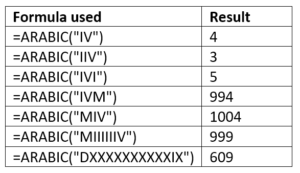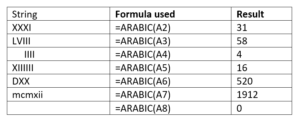# ARABIC Function

Converts a Roman numeral to Arabic numeral

## What is the ARABIC Function?

The ARABIC Function is an Excel Math and Trigonometry function. The function will convert a Roman numeral to Arabic numeral. It was introduced in MS Excel 2013.

### Syntax

=ARABIC(text)

Where:

Text (required argument) – The text is a string enclosed in quotation marks. It can also be an empty string (“”) or a reference to a cell containing the string.

### How to use the ARABIC Function in Excel?

To understand the uses of this function, let’s consider a few examples:

#### Example 1

If we provide the string as IV, the ARABIC function will return the value 4. The formula to be used is =ARABIC(“IV”).

Similarly, below are a few more examples:#### Example 2

We can also provide a reference to the cell containing the string. For example, as shown below, we provided a cell reference:As seen above:

• An empty string returns 0.
• The space before and after the string is ignored.
• When giving a cell reference, we do not need to use quotation marks.

### Things to remember about the ARABIC Function

1. #VALUE! error – Occurs when the given value is an invalid value. So, this error will occur when the numbers, dates, and text are not valid Roman numerals.
2. #NAME? error – Occurs when we provide the string without “”.
3. When we use an empty string as an input value in this function, that is (“”), then Excel returns 0 for it.
4. The function accepts 255 characters, which is the maximum length of the argument. So, the largest number that can be returned is 255,000.
5. It is indifferent to the case of the text. So, a text entered in UPPER case of the text argument will give the same result as the text entered in lowercase. For example, MXMV will return the same result as mxmv – 1995.
6. The function ignores leading and trailing spaces. For example, =ARABIC(” MXMV”) will give same result as =ARABIC(“MXMV”).
7. It supports the evaluation of a negative Roman numeral, even though a negative Roman number is non-standard. For this, we need to insert a negative sign before the Roman text. For example, =ARABIC(“-MXMV”) = -1995.
8. The ARABIC function is the opposite of the ROMAN Function.

Thanks for reading CFI’s guide to important Excel functions! By taking the time to learn and master these functions, you’ll significantly speed up your financial analysis. To learn more, check out these additional CFI resources:

• Excel Functions for Finance